SSC  >  Logical Reasoning - Test 4

# Logical Reasoning - Test 4

Test Description

## 25 Questions MCQ Test SSC CGL Tier 1 Mock Test Series | Logical Reasoning - Test 4

Logical Reasoning - Test 4 for SSC 2022 is part of SSC CGL Tier 1 Mock Test Series preparation. The Logical Reasoning - Test 4 questions and answers have been prepared according to the SSC exam syllabus.The Logical Reasoning - Test 4 MCQs are made for SSC 2022 Exam. Find important definitions, questions, notes, meanings, examples, exercises, MCQs and online tests for Logical Reasoning - Test 4 below.
Solutions of Logical Reasoning - Test 4 questions in English are available as part of our SSC CGL Tier 1 Mock Test Series for SSC & Logical Reasoning - Test 4 solutions in Hindi for SSC CGL Tier 1 Mock Test Series course. Download more important topics, notes, lectures and mock test series for SSC Exam by signing up for free. Attempt Logical Reasoning - Test 4 | 25 questions in 15 minutes | Mock test for SSC preparation | Free important questions MCQ to study SSC CGL Tier 1 Mock Test Series for SSC Exam | Download free PDF with solutions
 1 Crore+ students have signed up on EduRev. Have you?
Logical Reasoning - Test 4 - Question 1

### In the following question, select the odd word from the given alternatives.

Detailed Solution for Logical Reasoning - Test 4 - Question 1

Pituitary, Pineal and Thyroid are glands whereas Thalamus is a part of a Brain and not a gland.

Logical Reasoning - Test 4 - Question 2

### In the following question, select the odd number pair from the given alternatives.

Detailed Solution for Logical Reasoning - Test 4 - Question 2

Here 2nd number is twice the number of the square of 1st number
1) 6 : 72 = 6 × 6 = 36 × 2 = 72
2) 9 : 162 = 9 × 9 = 81 × 2 = 162
3) 12 : 288 = 12 × 12 = 144 × 2 = 288
4) 16 : 476 = 16 × 16 = 256 × 2 = 512 which is not equal to 476
Hence 16 : 476 is the odd number pair

Logical Reasoning - Test 4 - Question 3

### In question given below find the odd one form the given responses.

Detailed Solution for Logical Reasoning - Test 4 - Question 3

Here in every option number of triangles and cross signs are same except in option 4 where number of triangles is less than number of cross sign.
Hence option 4 is correct option

Logical Reasoning - Test 4 - Question 4

Last Saturday was on the twentieth of the month. If Tuesday is 3 days ahead, what day of the week is today?

Detailed Solution for Logical Reasoning - Test 4 - Question 4

Saturday was on 20th of this month.
Tuesday is three days ahead means today is Saturday.

Logical Reasoning - Test 4 - Question 5

If ‘-‘ stands for ‘÷’, ‘+’ stands for ‘x’, ‘÷’ stands for ‘-‘ and ‘x’ stands for ‘+’, find out which one of the following is correct?

Detailed Solution for Logical Reasoning - Test 4 - Question 5

Rewriting the equation as per the given question
1) 42 × 6 ÷ 3 + 5 – 8 = 81 which is not equal to 80
2) 42 ÷ 6 × 3 – 5 + 8 = 24 which is equal to 24
3) 42 + 6 × 3 – 5 ÷ 8 = 59.3 which is not equal to 55
4) 42 – 6 + 3 × 5 ÷ 8 = 37.8 which is not equal to 39
Hence option 2 is the correct option.

Logical Reasoning - Test 4 - Question 6

In the questions given below, find out the missing number from the given responses?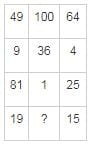Detailed Solution for Logical Reasoning - Test 4 - Question 6

Here the sum of square root of each number is written in the last column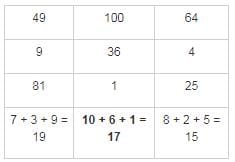Hence 17 is the missing number

Logical Reasoning - Test 4 - Question 7

Arrange the given words in the order in which they will be arranged in a dictionary and choose the one that comes second.

Detailed Solution for Logical Reasoning - Test 4 - Question 7

After arranging them as per the ascending order of dictionary we get;
Mirage, Misogamy, Misogynist, Misology

Logical Reasoning - Test 4 - Question 8

In a certain way, 'SIGHT' is related to EYES as 'FEEL' is related to –

Detailed Solution for Logical Reasoning - Test 4 - Question 8

EYES is used for SIGHT. Similarly, SKIN is used to FEEL.
Hence, “Skin” is the answer.

Logical Reasoning - Test 4 - Question 9

In the following question, select the related word from the given alternatives.

Anaemia : Blood ∷ Osteoporosis: ?

Detailed Solution for Logical Reasoning - Test 4 - Question 9

Anaemia is caused when there is a loss of blood in the body and ​Osteoporosis is
a disease where increased bone weakness increases the risk of a broken bone.

Logical Reasoning - Test 4 - Question 10

In the following question, select the related word from the given alternatives.

West : North – East ∷ South : ?

Detailed Solution for Logical Reasoning - Test 4 - Question 10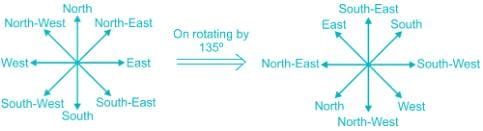Similarly, if we rotate direction indicator through 1350 in anti-clockwise direction then pointer indicating South would show North – West direction.

Logical Reasoning - Test 4 - Question 11

In the question, select the related number from the given alternatives.
7584 : 4251 ∷ 4563 : ?

Detailed Solution for Logical Reasoning - Test 4 - Question 11

In 7584 : 4251;
7 – 3 = 4; 5 – 3 = 2; 8 – 3 = 5; 4 – 3 = 1
Similarly;
4 – 3 = 1; 5 – 3 = 2; 6 – 3 = 3; 3 – 3 = 0Hence, “1230” is correct option.

Logical Reasoning - Test 4 - Question 12

Find the missing letter from the given responses.

AHN, BIO, CJP,?

Detailed Solution for Logical Reasoning - Test 4 - Question 12

A + 1 = B; B + 1 = C; C + 1 = D
H + 1 = I; I + 1 = J; J + 1 = K
N + 1 = O; O + 1 = P; P + 1 = Q
Hence the option “DKQ” is correct.

Logical Reasoning - Test 4 - Question 13

Find the missing number from the given responses.

9, 25, 49, 81, 121,?

Detailed Solution for Logical Reasoning - Test 4 - Question 13

Here the logic is square of every odd number starting from 3
9 = 3 × 3
25 = 5 × 5
49 = 7 × 7
81 = 9 × 9
121 = 11 × 11
169 = 13 × 13
Hence 169 is the missing number.

Logical Reasoning - Test 4 - Question 14

Asha is Bijay’s sister, Chitra is Bijay’s mother, Dev is Chitra’s father, Elina is Dev’s mother, then how Asha is related to Dev?

Detailed Solution for Logical Reasoning - Test 4 - Question 14

Table is drawn describing symbols and their meanings: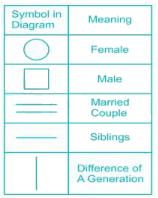family chart can be represented as:-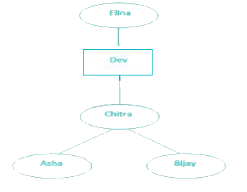It is clear from the chart that Asha is Dev’s granddaughter.

Logical Reasoning - Test 4 - Question 15

In the following question number of letters skipped in between adjacent letters in the series increases one each time. Which of the following series observe the rule?

Detailed Solution for Logical Reasoning - Test 4 - Question 15

1. RTWAG = R + 2 = T; T + 3 = W; W + 4 = A; A + 6 = G
2. EGJNR = E + 2 = G; G + 3 = J; J + 4 = N; N + 4 = R
3. NOQUZ = N + 1 = O; O + 2 = Q; Q + 4 = U;U + 5 = Z
4. CEHLQ = C + 2 = E; E + 3 = H; H + 4 = L; L + 5 = Q
Only “CEHLQ” follows the given rule.

Logical Reasoning - Test 4 - Question 16

Identify the missing number in the following figures?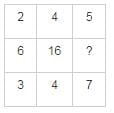Detailed Solution for Logical Reasoning - Test 4 - Question 16

Here 1st and 3rd number of each row in multiplied and result is written in the middle column.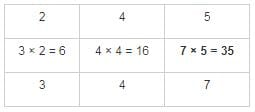Hence 35 is the missing number

Logical Reasoning - Test 4 - Question 17

A statement has been given, which is followed by four inferences marked (1), (2), (3) and (4) in the options. Find out the inference that definitely follows.
Most dresses in that shop are expensive.
Inferences:

Detailed Solution for Logical Reasoning - Test 4 - Question 17

1. All dresses in that shop are expensive => can be partially true
2. There are cheap dresses also in that shop =>  since most dresses are expensive, not all of them are so.
This means some cheap dresses are also there in the shop.
3. Handloom dresses in that shop are cheap => information is not given about handloom dresses
4. There are no cheap dresses available in that shop => information regarding non availability of cheap dresses is also not given
Hence only option 2 definitely follows the interference.

Logical Reasoning - Test 4 - Question 18

In a certain code language, if the word ‘RHOMBUS’ is code as TJQODWU, then how is the word ‘RECTANGLE’ in that language?

Detailed Solution for Logical Reasoning - Test 4 - Question 18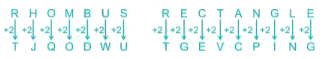As ‘RHOMBUS’ is codded as TJQODWUthen‘RECTANGLE’ is coded as TGEVCPING.

Logical Reasoning - Test 4 - Question 19

If in a certain code “Education” is written as “365798214” then how “Conduct” can be written as?

Detailed Solution for Logical Reasoning - Test 4 - Question 19

Here, “Education” ⇒ “365798214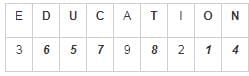Therefore “CONDUCT”  “7146578”

Logical Reasoning - Test 4 - Question 20

Hospital is 12 km toward east of Ankit’s house. His school is 5 km toward south of hospital. What is the shortest distance between Ankit’s house and school?

Detailed Solution for Logical Reasoning - Test 4 - Question 20

Direction path can be traced as: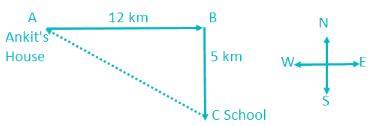⇒ AC = √[(AB)2 + (BC)2]
⇒ AC = √[(12)2 + (5)2]
⇒ AC = √[144 + 25]
⇒ AC = √169
⇒ AC = 13 km
Hence, Shortest distance between Ankit house and School is 13 km.

Logical Reasoning - Test 4 - Question 21

A word is represented by only one set of numbers as given in any one of the alternatives. The sets of numbers given in the alternatives are represented by two classes of alphabets as in two matrices given below. The columns and rows of Matrix I are numbered from 0 to 4 and that of Matrix II are numbered from 5 to 9. A letter from these matrices can be represented first by its row and next by its columne.g.‘M’ can be represented by 0114 etc.and ‘S’ can be represented by 5877 etc. Similarlyyou have to identify the set for the word ‘ROHAN’.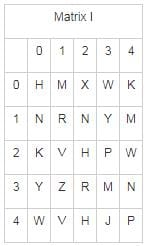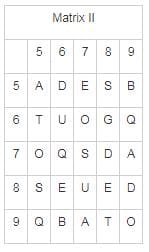Detailed Solution for Logical Reasoning - Test 4 - Question 21

1) 11, 75, 00, 55, 10 ⇒ R, O, H, A, N
2) 1157005512 ⇒ REHAN
3) 3267415512 ⇒ ROVAN
4) 3275215510 ⇒ ROVAN
Hence, 11, 75, 00, 55, 10numbers represent “ROHAN”.

Logical Reasoning - Test 4 - Question 22

From the given alternatives wordsselect the word which cannot be formed using the letters of the given word.

KILOMETERS

Detailed Solution for Logical Reasoning - Test 4 - Question 22

“STREET” cannot be formed from “KILOMETERS”as there are only one T in KILOMETERS but street has two T.

Logical Reasoning - Test 4 - Question 23

Directions: In the question one/two statements are given, followed by two conclusions/assumptions, I and II. You have to consider the statements to be true even if they seem to be at variance from commonly known facts. You have to decide which of the given conclusion/assumptions, if any, follows from the given statements.

Statements:
1. All student are girls.
2. Some students are not – talented.

Conclusion:
I. No student is talented.
II. Some girls are not – talented.

Detailed Solution for Logical Reasoning - Test 4 - Question 23

The Venn diagram of given statement can be represented as shown below: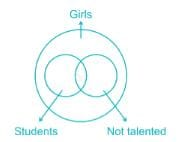I. No student is talented. ⇒ False
II. Some girls are not – talented. ⇒ True (as shown in diagram)
Hence, only conclusion II follows

Logical Reasoning - Test 4 - Question 24

Which of the following figure represent the relationship between:

Zebra, Grass eating animal, Lion?

Detailed Solution for Logical Reasoning - Test 4 - Question 24

Zebra, Grass eating animal, Lion
1. All Zebras are Grass-eating animals.
2. Not all Grass-eating animals are Zebras.
3. No Grass-easting animal is Lion.
4. No Zebra is Lion.
These 4 points can be represented as: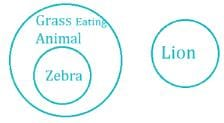Logical Reasoning - Test 4 - Question 25

Which answer figure will complete the pattern in the question figure?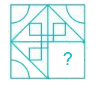Detailed Solution for Logical Reasoning - Test 4 - Question 25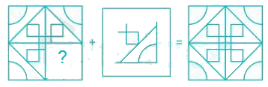Hencediagram in option 2) will complete the pattern

## SSC CGL Tier 1 Mock Test Series

42 docs|103 tests
 Use Code STAYHOME200 and get INR 200 additional OFF Use Coupon Code
Information about Logical Reasoning - Test 4 Page
In this test you can find the Exam questions for Logical Reasoning - Test 4 solved & explained in the simplest way possible. Besides giving Questions and answers for Logical Reasoning - Test 4, EduRev gives you an ample number of Online tests for practice

## SSC CGL Tier 1 Mock Test Series

42 docs|103 tests